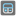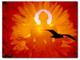• # New mission series "Calculator"!

## Question related to missionCalculator-VII

In this series of missions you are going to build an elementary calculator.

As an input, you get a sequence (string) of keys pressed , and, as the result of that function (string), you should show what will be shown on the screen when the last key is pressed .

Calculator-I - In the first mission only digits and single signs (only +, -, =) between them are used.

Calculator-II - In this mission the combinations of signs are presented.

Calculator-III - Max digit space of 5 is added. It means that the maximum number you can enter is "99999".

Calculator-IV - In the forth mission float numbers are allowed. The "." takes space as one digit, so max digit space is 4 for float number results. It means that the maximum float number you can enter is "9999.".

Calculator-V - In the fifth mission your function should work properly with additional operations: , /, // (integer division), % (modulo) and * (power) and their combinations with "=".

Calculator-VI - In the sixth mission are added such buttons as capital "B" (backspace) - delete previous character and capital "C" (clean) - delete all output, including error.

Calculator-VII - In the seventh mission the memory should be implemented with such operations: capital "A" (add, +), capital "S" (subtract, -), capital "G" (get value) and capital "E" (erase memory).

Good luck!36